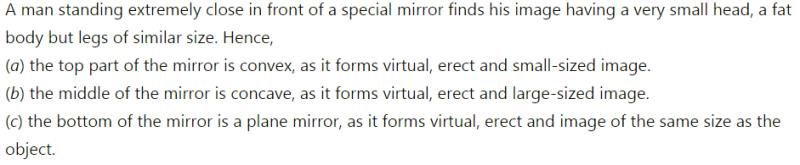Courses

# Test: Spherical Mirror

## 5 Questions MCQ Test | Test: Spherical Mirror

Description
This mock test of Test: Spherical Mirror for Class 10 helps you for every Class 10 entrance exam. This contains 5 Multiple Choice Questions for Class 10 Test: Spherical Mirror (mcq) to study with solutions a complete question bank. The solved questions answers in this Test: Spherical Mirror quiz give you a good mix of easy questions and tough questions. Class 10 students definitely take this Test: Spherical Mirror exercise for a better result in the exam. You can find other Test: Spherical Mirror extra questions, long questions & short questions for Class 10 on EduRev as well by searching above.
QUESTION: 1

### A spherical mirror in which silver coating is done on the inner surface is

Solution:

Convex Mirror is a curved mirror where the reflective surface bulges out towards the light source. Silver coating is done inside the mirror.

QUESTION: 2

### Which mirror is used to concentrate the light on a given spot ?

Solution:

A concave mirror, or converging mirror, has a reflecting surface that is recessed inward. Concave mirrors reflect light inward to one focal point, they are used to focus light.

QUESTION: 3

### A man standing in front of a special mirror finds his image having a very small head, a fat body and legs of normal size. The shapes of the three parts of the special mirror are

Solution:QUESTION: 4

The name and radius of curvature of a spherical mirror whose focal length is +2 cm is

Solution:

Radius of curvature ( R) = 2* Focal length (f)
Since, f=+2 cm, therefore, R= 2*(+2) = +4 cm
If it is a convex mirror, since it's focal length is positive.

QUESTION: 5

The concave mirrors are used in

Solution:

A reflecting telescope uses concave mirror as they produce images similar to the images produce by convex lenses ie. , upright and smaller than the  object.
The magic lantern uses a concave mirror in back of a light source to direct as much of the light as possible through a small rectangular sheet of glass.
Concave mirrors project a beam of light outward, which is required in a cinema projector.